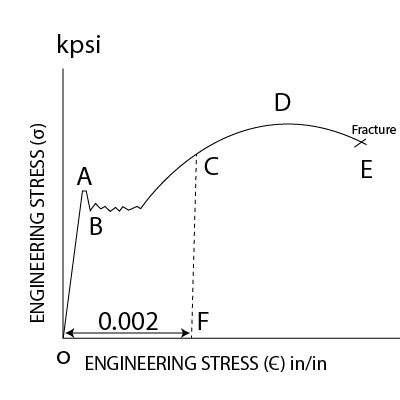# The following is an engineering stress (?) versus engineering strain (?) curve for an engineering...

## Question:

The following is an engineering stress ({eq}\sigma {/eq}) versus engineering strain ({eq}\epsilon {/eq}) curve for an engineering material. The stress and strain at points A, B, C, D, E are {eq}(\sigma_ e = 38 \ kpsi, \epsilon_e =0.0013), (\sigma_ e =36 kpsi, \epsilon_e =0.00131), (\sigma_ e =52 kpsi, \epsilon_e =0.0038), (\sigma_ e=63 kpsi, \epsilon_e =0.2), and (\sigma_ e =54 kpsi, \epsilon_e =0.3) {/eq}, respectively. CF line is parallel to the AO line.

Determine the upper yield strength, lower yield strength, modulus of elasticity, 0.2% offset yield strength, ultimate tensile strength, the amount of elastic strain and plastic strain at point C. Also, determine the true stress and true strain at point D.## Hooke's Law:

The stress generated in the material of concern has a proportional fashion dependency on the quantity of strain generated. This statement is what is widely referred to as Hooke's law. This proportionality is replaced by a constant, which is designated as modulus of elasticity. A material shows a negligible amount of plastic strain generated in this region of proportional dependency on a stress-strain curve.

## Answer and Explanation:

Become a Study.com member to unlock this answer! Create your account

Given Data

• The engineering stress at point A is: {eq}{\sigma _{eA}} = 38\;{\rm{kpsi}} {/eq} .
• The engineering strain at point A is:...

See full answer below.

#### Learn more about this topic:Hooke's Law & the Spring Constant: Definition & Equation

from

Chapter 4 / Lesson 19
199K

After watching this video, you will be able to explain what Hooke's Law is and use the equation for Hooke's Law to solve problems. A short quiz will follow.